### IMO Shortlist 1991 problem 28

Kvaliteta:
Avg: 0.0
Težina:
Avg: 0.0
We call a set$S$ on the real line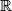$\mathbb{R}$ superinvariant if for any stretching$A$ of the set by the transformation taking$x$ to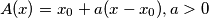$A(x) = x_0 + a(x - x_0), a > 0$ there exists a translation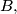$B,$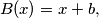$B(x) = x+b,$ such that the images of$S$ under$A$ and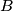$B$ agree; i.e., for any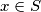$x \in S$ there is a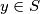$y \in S$ such that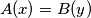$A(x) = B(y)$ and for any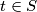$t \in S$ there is a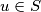$u \in S$ such that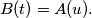$B(t) = A(u).$ Determine all superinvariant sets.
Source: Međunarodna matematička olimpijada, shortlist 1991